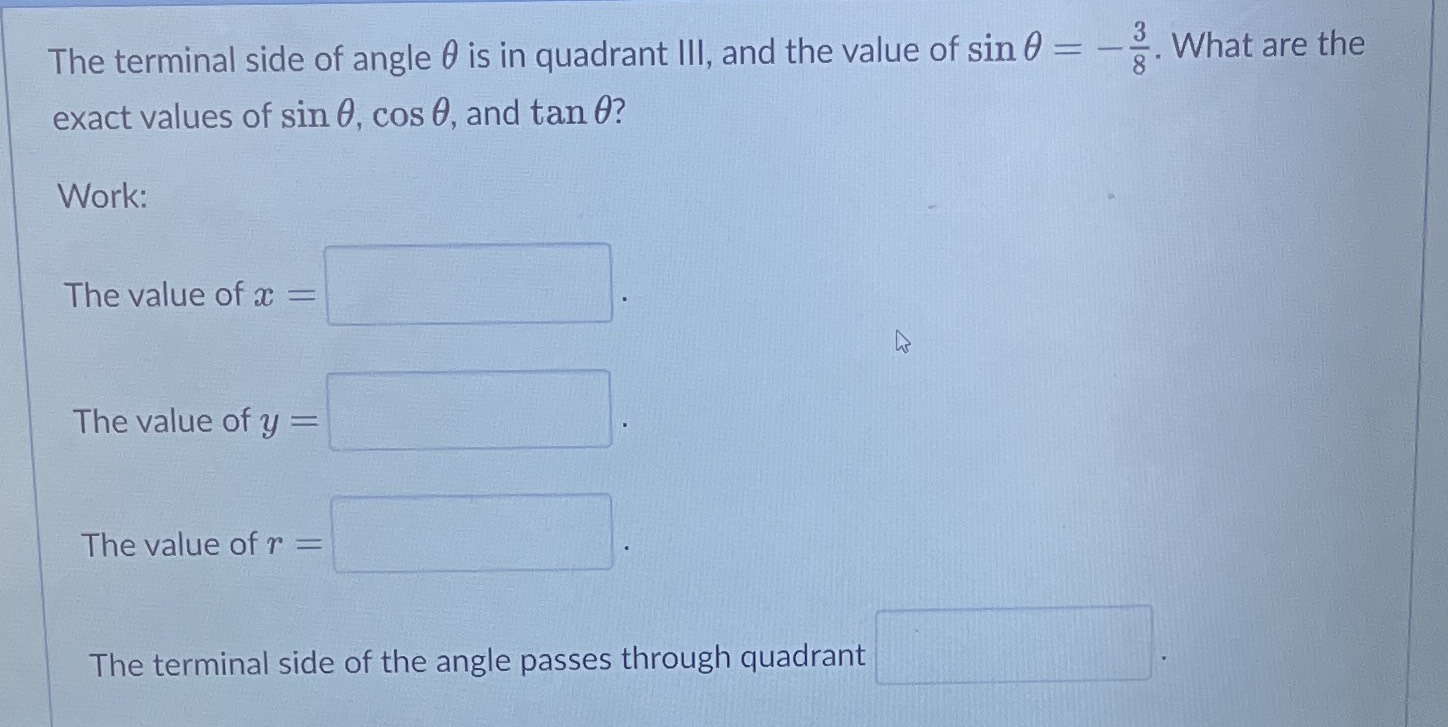### Still have math questions?

Trigonometry
QuestionThe terminal side of angle $$\theta$$ is in quadrant III, and the value of $$\sin \theta = - \frac { 3 } { 8 }$$ . What are the exact values of $$\sin \theta , \cos \theta ,$$ and $$\tan \theta$$ ? Work:

The value of $$x =$$

The value of $$y =$$

The value of $$r =$$

The terminal side of the angle passes through quadrant

-$$\sqrt{55}$$